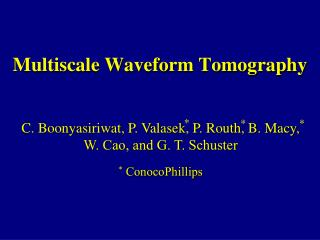DownloadDownload PresentationMultiscale Waveform Tomography

# Multiscale Waveform Tomography

Télécharger la présentation## Multiscale Waveform Tomography

- - - - - - - - - - - - - - - - - - - - - - - - - - - E N D - - - - - - - - - - - - - - - - - - - - - - - - - - -
##### Presentation Transcript

1. Multiscale Waveform Tomography * * * C. Boonyasiriwat, P. Valasek, P. Routh, B. Macy, W. Cao, and G. T. Schuster * ConocoPhillips

2. Outline • Goal • Introduction • Theory of Acoustic Waveform Tomography • Multiscale Waveform Tomography • Results • Conclusions 1

3. Goal 2

4. Outline • Goal and Motivation • Introduction • Theory of Acoustic Waveform Tomography • Multiscale Waveform Tomography • Results • Conclusions 3

5. Introduction ? 4

6. Introduction: Waveform Tomography • No high frequency approximation. • Frequency domain: Pratt et al. (1998), etc. • Time domain: Zhou et al. (1995), Sheng et al. (2006), etc. • Pratt and Brenders (2004) and Sheng et al. (2006) used early-arrival wavefields. • Bunks et al. (1995) and Pratt et al. (1998) used multiscale approaches. 9

7. Outline • Goal • Introduction • Theory of Acoustic Waveform Tomography • Multiscale Waveform Tomography • Results • Conclusions 10

8. Why Acoustic? • Elastic wave equation is expensive. • Waveform inversion is also expensive. • Previous research shows acoustics is adequate. • Use acoustics and mute unpredicted wavefields. 11

9. Theory of Waveform Tomography The waveform misfit function is An acoustic wave equation: 12

10. Theory of Waveform Tomography The steepest descent method can be used to minimize the misfit function: The waveform residual is defined by 13

11. Theory of Waveform Tomography where The gradient is calculated by 14

12. Outline • Goal • Introduction • Theory of Acoustic Waveform Tomography • Multiscale Waveform Tomography • Results • Conclusions 15

13. Why Use Multiscale? Misfit function ( f ) Model parameter (m) Low Frequency Coarse Scale High Frequency Fine Scale Image from Bunks et al. (1995) 16

14. Our Multiscale Approach • Combine Early-arrival Waveform Tomography (Sheng et al., 2006) and a time-domain multiscale approach (Bunks et al., 1995). • Use a Wiener filter for low-pass filtering the data. • Use a window function to mute all energy except early arrivals. • Use multiscale V-cycles. 17

15. Why a Wiener Filter? Target Wavelet Original Wavelet Wavelet: Hamming Window Wavelet: Wiener Filter 18

16. Multiscale V-Cycle High Frequency Fine Grid Low Frequency Coarse Grid 19

17. Outline • Goal • Introduction • Theory of Acoustic Waveform Tomography • Multiscale Waveform Tomography • Results • Conclusions 20

18. Synthetic SSP Data Results • Layered Model with Scatterers • SEG Salt Model • Mapleton Model 21

20. EWT Tomogram using 15-Hz Data Gradient 25

21. MWT Tomogram using 2.5-Hz Data Gradient 26

22. MWT Tomogram using 5-Hz Data 2.5-Hz 27

23. MWT Tomogram using 15-Hz Data 10 Hz 29

24. Comparison of Misfit Function 15 Hz 15 Hz 5 Hz 10 Hz 2.5 Hz 31

26. MWT Tomogram (2.5,5 Hz) TRT 34

27. Marine Data 480 Hydrophones 515 Shots 12.5 m dt = 2 ms Tmax = 10 s 41

28. Waveform Residual vs Iteration Number 1 s 2 s 5 Hz 5 Hz 5 Hz 10 Hz 10 Hz 5 Hz 10 Hz 45

29. Common Image Gather 5 Hz 10 Hz 46

30. Outline • Goal • Introduction • Theory of Acoustic Waveform Tomography • Multiscale Waveform Tomography • Results • Conclusions 47

31. Conclusions • MWT partly overcomes the local minima problem. • MWT provides more accurate and highly resolved than TRT and EWT. • MWT is much more expensive than TRT. • Accuracy is more important than the cost. • MWT provides very accurate tomograms for synthetic data and shows encouraging results for the marine data. 48

32. Future Work • Use wider-window data and finally use all the data to obtain more accurate velocity distributions. • Take into account the source radiation pattern. • Apply MWT to land data. 49